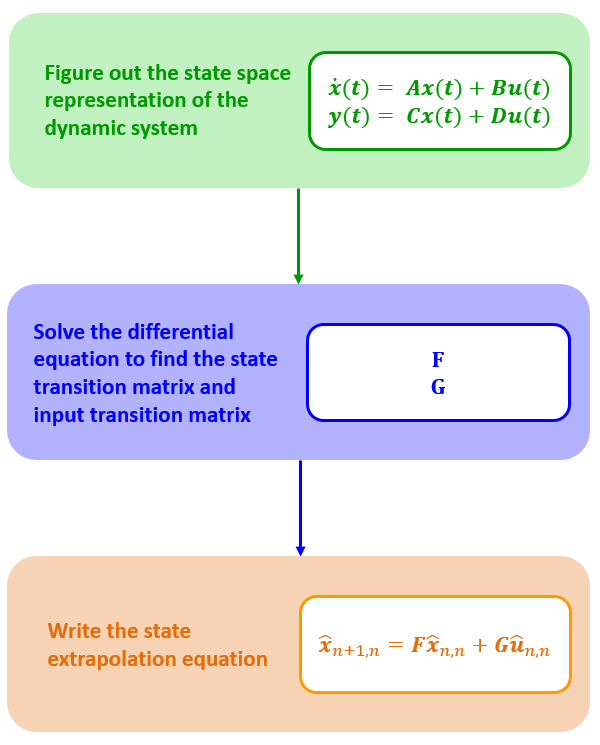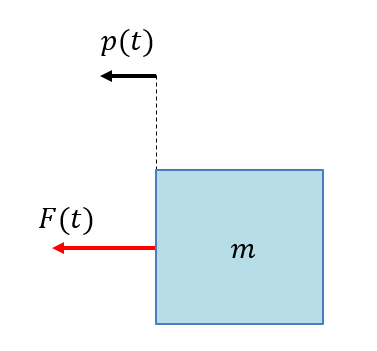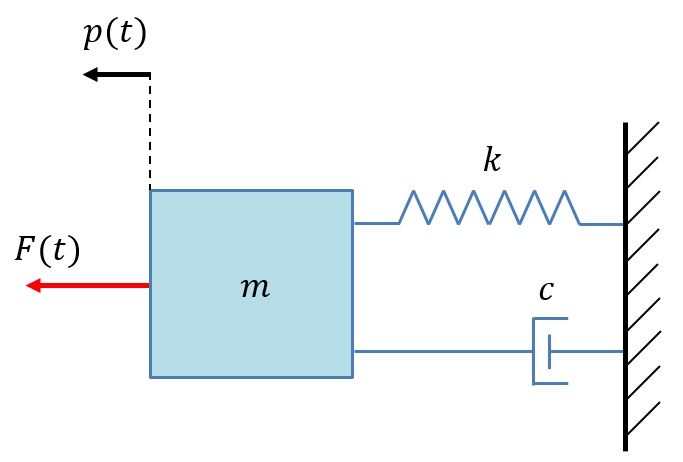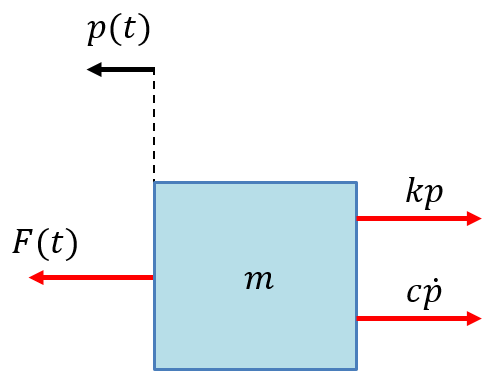﻿ Modeling linear dynamic system

# Modeling linear dynamic systems

Well, that was easy to describe a dynamic model for an airplane. I suppose that you are familiar with Newton's motion equations from high school.

This chapter generalizes dynamic model derivation for any linear dynamic system. The following description includes integrals and differential equations.

This chapter is the most challenging chapter of the tutorial. It is not required for the understanding of the Kalman Filter principles. If you feel uncomfortable with this math – feel free to skip this chapter.

On my side, I tried to make my explanations as straightforward and easy to follow as possible, and, of course, I provided real-life examples.

## Derivation of the state extrapolation equation

Our goal is to derive the state extrapolation equation in the form:

$\boldsymbol{\hat{x}}_{n+1,n} =\boldsymbol{F\hat{x}}_{n,n} + \boldsymbol{G\hat{u}}_{n,n} + \boldsymbol{w}_{n}$

To do that, we need to model the dynamic system. In other words, to figure out the state space representation of the dynamic system. The following two equations are the state-space representation of the LTI system:

$\boldsymbol{\dot{x}(t) = Ax(t) + Bu(t)}$ $\boldsymbol{y(t) = Cx(t) + Du(t)}$
Where:
 $$\boldsymbol{x}$$ is the state vector $$\boldsymbol{y}$$ is the output vector $$\boldsymbol{A}$$ is the system's dynamics matrix $$\boldsymbol{B}$$ is the input matrix $$\boldsymbol{C}$$ is the output matrix $$\boldsymbol{D}$$ is the feedthrough matrix

To find the state transition matrix $$\boldsymbol{F}$$ and input transition matrix $$\boldsymbol{G}$$, we need to solve the state space differential equation.

The following diagram summarizes the process of the state extrapolation equation derivation.## The state space representation

You might be wondering why the state space representation must be in the following form:

$\boldsymbol{\dot{x}(t) = Ax(t) + Bu(t)}$ $\boldsymbol{y(t) = Cx(t) + Du(t)}$

Many computer software packages that solve differential equations require this representation.

The best way to describe the state space representation is by examples.

### Example - constant velocity moving body

Since there is no external force applied to the body, the system has no inputs:

$\boldsymbol{u(t)} = 0$

The state space variable $$\boldsymbol{x(t)}$$ is the body's displacement $$p(t)$$ and the speed $$v(t)$$.

$\boldsymbol{x(t)} = \left[ \begin{matrix} p\\ v\\ \end{matrix} \right]$

Note: To avoid confusion between the state vector $$\boldsymbol{x(t)}$$ (bold-face font) and the body position along the $$x$$ - axis denoted by $$x(t)$$ (normal-face font), I've denoted the body position by $$p(t)$$.

$\boldsymbol{\dot{x}(t) = Ax(t) + Bu(t)}$

$\left[ \begin{matrix} \dot{p}\\ \dot{v}\\ \end{matrix} \right] = \boldsymbol{A} \left[ \begin{matrix} p\\ v\\ \end{matrix} \right] + \boldsymbol{B} \times 0$

$\left[ \begin{matrix} \dot{p}\\ \dot{v}\\ \end{matrix} \right] = \left[ \begin{matrix} 0 & 1\\ 0 & 0\\ \end{matrix} \right] \left[ \begin{matrix} p\\ v\\ \end{matrix} \right]$

$\boldsymbol{A} = \left[ \begin{matrix} 0 & 1\\ 0 & 0\\ \end{matrix} \right]$

We've got the first equation in a differential form.

The dynamic system output $$\boldsymbol{y(t)}$$ is the body displacement $$\boldsymbol{p(t)}$$ that is also the state variable.

$\boldsymbol{y(t) = Cx(t) + Du(t)}$

$p = \boldsymbol{C} \left[ \begin{matrix} p\\ v\\ \end{matrix} \right] + \boldsymbol{D} \times 0$

$p = \left[ \begin{matrix} 1 & 0\\ \end{matrix} \right] \left[ \begin{matrix} p\\ v\\ \end{matrix} \right]$

$\boldsymbol{C} = \left[ \begin{matrix} 1 & 0\\ \end{matrix} \right]$

We are done.

### Modeling high-order dynamic systems

Many dynamic systems models are described by high-order differential equations. The order of the differential equation is the number of the highest derivative in a differential equation.

To tackle a high-order equation, we should reduce it to the first-order differential equation by defining new variables and substituting them into the highest-order terms.

#### The algorithm for reducing the differential equation order

A general $$n$$-th order linear differential equation can be expressed as a system of $$n$$ first-order differential equations.

The high-order governing equation of the dynamic system looks as follows:

$a_{n}\frac{d^{n}y}{dt^{n}}+ a_{n-1}\frac{d^{n-1}y}{dt^{n-1}}+ \ldots + a_{2}\frac{d^{2}y}{dt^{2}}+a_{1}\frac{d^{1}y}{dt^{1}}+ a_{0}y = u$

The governing equation completely characterizes the dynamic state of the system.

Reducing the equation order:

1. Isolate the highest-order derivative:
2. $\frac{d^{n}y}{dt^{n}}= -\frac{a_{0}}{a_{n}}y- \frac{a_{1}}{a_{n}}\frac{d^{1}y}{dt^{1}}- \frac{a_{2}}{a_{n}}\frac{d^{2}y}{dt^{2}}- \ldots - \frac{a_{n-1}}{a_{n}}\frac{d^{n-1}y}{dt^{n-1}}+\frac{1}{a_{n}}u$

$$y$$ and its first $$n - 1$$ derivatives are the states of this system.

3. Define new variables:
4. Setting $$x_{1} = y$$, we write:

$x_{1} \left( t \right) =y$ $x_{2} \left( t \right) = \frac{dy}{dt}$ $x_{3} \left( t \right) = \frac{d^{2}y}{dt^{2}}$ $\vdots$ $x_{n} \left( t \right) = \frac{d^{n-1}y}{dt^{n-1}}$

Now, functions $$x_{i}(t)$$ are the state variables.

5. Take the derivatives of the state variables:
6. $\frac{dx_{1}}{dt}=x_{2} \left( t \right)$ $\frac{dx_{2}}{dt}=x_{3} \left( t \right)$ $\vdots$ $\frac{dx_{n-1}}{dt}=x_{n} \left( t \right)$ $\frac{dx_{n}}{dt}=\frac{d^{n}y}{dt^{n}}$

7. Plug the isolated $$\frac{d^{n}y}{dt^{n}}$$ term (see step 1) into the last equation:
8. $\frac{dx_{1}}{dt}=x_{2} \left( t \right)$ $\frac{dx_{2}}{dt}=x_{3} \left( t \right)$ $\vdots$ $\frac{dx_{n-1}}{dt}=x_{n} \left( t \right)$ $\frac{dx_{n}}{dt}=-\frac{a_{0}}{a_{n}}x_{1}- \frac{a_{1}}{a_{n}}x_{2}- \frac{a_{2}}{a_{n}}x_{3}- \ldots - \frac{a_{n-1}}{a_{n}}x_{n}+\frac{1}{a_{n}}u$

9. Express the resulting system of equations using vector-matrix notation:
10. $\left[ \begin{matrix} \frac{dx_{1}}{dt}\\ \frac{dx_{2}}{dt}\\ \vdots \\ \frac{dx_{n-1}}{dt}\\ \frac{dx_{n}}{dt}\\ \end{matrix} \right] = \left[ \begin{matrix} \dot{x}_{1}\\ \dot{x}_{2}\\ \vdots \\ \dot{x}_{n-1}\\ \dot{x}_{n}\\ \end{matrix} \right] = \left[ \begin{matrix} 0 & 1 & 0 & \cdots & 0 & 0\\ 0 & 0 & 1 & \cdots & 0 & 0\\ \vdots & \vdots & \vdots & \ddots & \vdots & \vdots \\ 0 & 0 & 0 & \cdots & 0 & 1\\ -\frac{a_{0}}{a_{n}} & -\frac{a_{1}}{a_{n}} & -\frac{a_{2}}{a_{n}} & \cdots & -\frac{a_{n-2}}{a_{n}} & -\frac{a_{n-1}}{a_{n}}\\ \end{matrix} \right] \left[ \begin{matrix} x_{1}\\ x_{2}\\ \vdots \\ x_{n-1}\\ x_{n}\\ \end{matrix} \right] + \left[ \begin{matrix} 0\\ 0\\ \vdots \\ 0\\ \frac{1}{a_{n}}\\ \end{matrix} \right] u$

That's it. We've got the state space equation in the form of the following:

$\boldsymbol{\dot{x}(t) = Ax(t) + Bu(t)}$

Where:

$\boldsymbol{A} = \left[ \begin{matrix} 0 & 1 & 0 & \cdots & 0 & 0\\ 0 & 0 & 1 & \cdots & 0 & 0\\ \vdots & \vdots & \vdots & \ddots & \vdots & \vdots \\ 0 & 0 & 0 & \cdots & 0 & 1\\ -\frac{a_{0}}{a_{n}} & -\frac{a_{1}}{a_{n}} & -\frac{a_{2}}{a_{n}} & \cdots & -\frac{a_{n-2}}{a_{n}} & -\frac{a_{n-1}}{a_{n}}\\ \end{matrix} \right]$

$\boldsymbol{B} = \left[ \begin{matrix} 0\\ 0\\ \vdots \\ 0\\ \frac{1}{a_{n}}\\ \end{matrix} \right]$

Let us see two examples.

### Example - constant acceleration moving body

In this example, there is an external force applied to the body.

The governing equation for a moving body with constant acceleration is Newton's second law:

$m\ddot{p} = F$

Where:

$$p$$ is the body position displacement

$$m$$ is the body mass

$$F$$ is the external force applied to the bodyWe are going to apply an order reduction algorithm to the governing equation.

1. Isolate the highest-order derivative:
2. $\ddot{p} = \frac{1}{m}F$

3. Define new variables $$x_{1}$$ and $$x_{2}$$:
4. $x_{1} = p$ $x_{2} = \dot{p}$

$$x_{1}$$ and $$x_{2}$$ are the state variables.

5. Take the derivatives of the state variables:
6. $\dot{x}_{1} = x_{2}$ $\dot{x}_{2} = \ddot{p}$

7. Plug in the isolated $$\ddot{p}$$ term (see step 1) in the last equation:
8. $\dot{x}_{1} = x_{2}$ $\dot{x}_{2} = \frac{F}{m}$

9. Express the resulting system of equations using vector-matrix notation:
10. $\left[ \begin{matrix} \dot{x}_{1}\\ \dot{x}_{2}\\ \end{matrix} \right] = \left[ \begin{matrix} 0 & 1\\ 0 & 0\\ \end{matrix} \right] \left[ \begin{matrix} x_{1}\\ x_{2}\\ \end{matrix} \right] + \left[ \begin{matrix} 0\\ \frac{1}{m}\\ \end{matrix} \right] F$

Remember that $$x_{2} = \dot{p}$$. It would be more meaningful to denote $$x_{2}$$ by $$v$$, since $$x_{2}$$ is the body velocity.

We can rewrite the above equation as follows:

$\left[ \begin{matrix} \dot{p}\\ \dot{v}\\ \end{matrix} \right] = \left[ \begin{matrix} 0 & 1\\ 0 & 0\\ \end{matrix} \right] \left[ \begin{matrix} p\\ v\\ \end{matrix} \right] + \left[ \begin{matrix} 0\\ \frac{1}{m}\\ \end{matrix} \right] F$

Or:

$\left[ \begin{matrix} \dot{p}\\ \dot{v}\\ \end{matrix} \right] = \left[ \begin{matrix} 0 & 1\\ 0 & 0\\ \end{matrix} \right] \left[ \begin{matrix} p\\ v\\ \end{matrix} \right] + \left[ \begin{matrix} 0\\ 1\\ \end{matrix} \right] a$

Where $$a$$ is the body acceleration resulting from the applied force $$F$$.

We've got an equation in the form:

$\boldsymbol{\dot{x}(t) = Ax(t) + Bu(t)}$

The state-space variable $$\boldsymbol{x(t)}$$ is the body's displacement $$p(t)$$ and the speed $$v(t)$$.

The dynamic system output $$\boldsymbol{y(t)}$$ is the body displacement $$p(t)$$ which is also an element of the state variable.

$\boldsymbol{y(t) = Cx(t) + Du(t)}$

$p = \boldsymbol{C} \left[ \begin{matrix} p\\ v\\ \end{matrix} \right] + \boldsymbol{D} a$

$p = \left[ \begin{matrix} 1 & 0\\ \end{matrix} \right] \left[ \begin{matrix} p\\ v\\ \end{matrix} \right] + \left[ \begin{matrix} 0\\ \end{matrix} \right] a$

### Example: mass-spring-damper system

The mass-spring-damper system includes three basic elements:

• mass - inertia element
• spring - elastic element
• damper - frictional elementEach of the elements has one of two possible energy behaviors:

• stores all the energy supplied to it
• dissipates all energy into heat by the “frictional” effect

The mass stores energy as kinetic energy.

When the spring is compressed from its original length, it stores the energy as potential energy.

The damper dissipates energy as a heat.

These three components: mass, spring, and damper, can model any dynamic response situation in a general sense.

The force diagram for this system is shown below.The spring force is proportional to the position displacement $$p$$ of the mass.

The viscous damping force is proportional to the velocity $$\dot{p}$$ of the mass.

Newton's second law states:

$\sum _{}^{}F = ma = m\frac{d^{2}p}{dt^{2}} = m\ddot{p}$

We proceed by summing the forces and applying Newton's second law:

$\sum_{}^{}F = F(t) - c\dot{p} - kp = m\ddot{p}$

Where:

$$p$$ is the body position displacement

$$m$$ is the body mass

$$F$$ is the external force applied to the body

$$k$$ is the spring constant

$$c$$ is the damping coefficient

This equation is a governing equation that completely characterizes the dynamic state of the system.

We are going to apply an order reduction algorithm to the governing equation.

1. Isolate the highest-order derivative:
2. $\ddot{p} = -\frac{k}{m}p - \frac{c}{m}\dot{p} + \frac{1}{m}F$

3. Define new variables $$x_{1}$$ and $$x_{2}$$:
4. $x_{1} = p$ $x_{2} = \dot{p}$

$$x_{1}$$ and $$x_{2}$$ are the state variables

5. Take the derivatives of the state variables:
6. $\dot{x}_{1} = x_{2}$ $\dot{x}_{2} = \ddot{p}$

7. Plug in the isolated $$\ddot{p}$$ term (see step 1) in the last equation:
8. $\dot{x}_{1} = x_{2}$ $\dot{x}_{2} = -\frac{k}{m}p - \frac{c}{m}\dot{p} + \frac{1}{m}F$

9. Express the resulting system of equations using vector-matrix notation:
10. $\left[ \begin{matrix} \dot{x}_{1}\\ \dot{x}_{2}\\ \end{matrix} \right] = \left[ \begin{matrix} 0 & 1\\ -\frac{k}{m} & -\frac{c}{m}\\ \end{matrix} \right] \left[ \begin{matrix} x_{1}\\ x_{2}\\ \end{matrix} \right] + \left[ \begin{matrix} 0\\ \frac{1}{m}\\ \end{matrix} \right] F$

Remember that: $$x_{2} = \dot{p}$$, It would be more meaningful to denote $$x_{2}$$ by $$v$$, since $$x_{2}$$ is the body velocity.

We can rewrite the above equation as follows:

$\left[ \begin{matrix} \dot{p}\\ \dot{v}\\ \end{matrix} \right] = \left[ \begin{matrix} 0 & 1\\ -\frac{k}{m} & -\frac{c}{m}\\ \end{matrix} \right] \left[ \begin{matrix} p\\ v\\ \end{matrix} \right] + \left[ \begin{matrix} 0\\ \frac{1}{m}\\ \end{matrix} \right] F$

We've got an equation in the form of the following:

$\boldsymbol{\dot{x}(t) = Ax(t) + Bu(t)}$

The state-space variable $$\boldsymbol{x(t)}$$ is the body's displacement $$p(t)$$ and the speed $$v(t)$$.

The dynamic system output $$\boldsymbol{y(t)}$$ is the body displacement $$p(t)$$ which is also an element of the state variable.

$\boldsymbol{y(t) = Cx(t) + Du(t)}$

$p = \boldsymbol{C} \left[ \begin{matrix} p\\ v\\ \end{matrix} \right] + \boldsymbol{D} F$

$p = \left[ \begin{matrix} 1 & 0\\ \end{matrix} \right] \left[ \begin{matrix} p\\ v\\ \end{matrix} \right] + \left[ \begin{matrix} 0\\ \end{matrix} \right] F$

### More examples

You can find many beautiful examples of state-space modeling on the ShareTechnote site.

## Solving the differential equation

Remember, for our Kalman Filter model, we need to determine the state extrapolation equation in the form of:

$\boldsymbol{\hat{x}}_{n+1,n} = \boldsymbol{F\hat{x}}_{n,n} + \boldsymbol{G\hat{u}}_{n,n}$

To get there, we shall solve the differential equation that describes the state space representation.

We can use computer software to solve the differential equation or do it ourselves.

Let us see how to solve the differential equation.

### Dynamic systems without input variable

LTI dynamic system without external input can be described by the first-order differential equation:

$\boldsymbol{\dot{x} = Ax}$

Where $$\boldsymbol{A}$$ is a system dynamics matrix.

Our goal is to find the state transition matrix $$\boldsymbol{F}$$.

We need to solve the differential equation to find $$\boldsymbol{F}$$.

In a single dimension, the differential equation looks as follows:

$\frac{dx}{dt} = kx$ $\frac{dx}{x} = kdt$

Integrating both sides results in the following:

$\int _{x_{0}}^{x_{1}}\frac{1}{x}dx = \int _{0}^{ \Delta t}kdt$

Solving the integrals:

$ln \left( x_{1} \right) - ln \left( x_{0} \right) =k \Delta t$ $ln \left( x_{1} \right) = ln \left( x_{0} \right) +k \Delta t$ $x_{1}= e^{ln \left( x_{0} \right) +k \Delta t}$ $x_{1}= e^{ln \left( x_{0} \right) }e^{k \Delta t}$ $x_{1}= x_{0}e^{k \Delta t}$

Similarly, in the multidimensional case, for:

$\boldsymbol{\dot{x} = Ax}$

The solution is:

$x_{n+1}= x_{n}e^{\boldsymbol{A} \Delta t}$

We've found the state transition matrix $$\boldsymbol{F}$$:

$\boldsymbol{F} = e^{\boldsymbol{A} \Delta t}$

$$e^{\boldsymbol{A}t}$$ is a matrix exponential.

The matrix exponential can be computed by Taylor series expansion:

$e^{\boldsymbol{X}}= \sum _{k=0}^{\infty}\frac{1}{k!}\boldsymbol{X}^{k}$

Therefore:

$\boldsymbol{F} = e^{\boldsymbol{A} \Delta t} = \boldsymbol{I} + \boldsymbol{A} \Delta t+ \frac{ \left( \boldsymbol{A} \Delta t \right) ^{2}}{2!}+ \frac{ \left( \boldsymbol{A} \Delta t \right) ^{3}}{3!}+ \frac{ \left( \boldsymbol{A} \Delta t \right) ^{4}}{4!}+ \ldots$

Where $$\boldsymbol{I}$$ is an identity matrix.

#### Example continued - constant velocity moving body

Now we can find the state transition matrix $$\boldsymbol{F}$$ for the constant velocity motion equations.

The following set of differential equations can describe the constant velocity dynamic model.

$\begin{cases} \frac{dp}{dt}=v\\ \frac{dv}{dt}=0\\ \end{cases}$

In a matrix form:

$\left[ \begin{matrix} \dot{p}\\ \dot{v}\\ \end{matrix} \right] = \left[ \begin{matrix} 0 & 1\\ 0 & 0\\ \end{matrix} \right] \left[ \begin{matrix} p\\ v\\ \end{matrix} \right]$ $\boldsymbol{A} = \left[ \begin{matrix} 0 & 1\\ 0 & 0\\ \end{matrix} \right]$

Let's calculate $$\boldsymbol{A}^{2}$$

$\boldsymbol{A}^{2} = \left[ \begin{matrix} 0 & 1\\ 0 & 0\\ \end{matrix} \right] \left[ \begin{matrix} 0 & 1\\ 0 & 0\\ \end{matrix} \right] = \left[ \begin{matrix} 0 & 0\\ 0 & 0\\ \end{matrix} \right]$

Since $$\boldsymbol{A}^{2} = 0$$, higher powers of $$\boldsymbol{A}$$ are also equal to 0.

Now, we can find the state transition matrix $$\boldsymbol{F}$$ for the constant velocity model:

$F= e^{\boldsymbol{A} \Delta t} = \boldsymbol{I} + \boldsymbol{A} \Delta t = \left[ \begin{matrix} 1 & 0\\ 0 & 1\\ \end{matrix} \right] + \left[ \begin{matrix} 0 & 1\\ 0 & 0\\ \end{matrix} \right] \Delta t = \left[ \begin{matrix} 1 & \Delta t\\ 0 & 1\\ \end{matrix} \right]$ $\boldsymbol{x}_{n+1} = \boldsymbol{F}\boldsymbol{x}_{n} = \left[ \begin{matrix} 1 & \Delta t\\ 0 & 1\\ \end{matrix} \right] \boldsymbol{x}_{n}$ $\left[ \begin{matrix} x_{n+1}\\ \dot{x}_{n+1}\\ \end{matrix} \right] = \left[ \begin{matrix} 1 & \Delta t\\ 0 & 1\\ \end{matrix} \right] \left[ \begin{matrix} x_{n}\\ \dot{x}_{n}\\ \end{matrix} \right]$

### Dynamic systems with an input variable

For zero-order hold sampling, assuming the input is piecewise constant, the general solution of the state space equation in the form of:

$\boldsymbol{\dot{x}(t) = Ax(t) + Bu(t)}$

is given by:

$\boldsymbol{x ( t+ \Delta t )} = \color{red}{\underbrace{e^{\boldsymbol{A} \Delta t}}_\textrm{F}} \boldsymbol{x(t)} + \color{blue}{\underbrace{\int _{0}^{\Delta t} e^{\boldsymbol{A}t}dt\boldsymbol{B}}_\textrm{G}} \boldsymbol{u(t)}$

If we remove the input variable $$(\boldsymbol{u(t)} = 0)$$, we get the solution derived in the previous chapter.

I am not going to prove this - you can find the proof in Tom M. Apostol's "Calculus" textbook (second edition), theorem 8.3, or any other calculus textbook.

Now let's solve the state space equations for the constant acceleration moving body and the mass-spring-damper system examples.

#### Example continued - constant acceleration moving body

Recall that the state-space representation of the constant acceleration moving body is:

$\left[ \begin{matrix} \dot{p}\\ \dot{v}\\ \end{matrix} \right] = \left[ \begin{matrix} 0 & 1\\ 0 & 0\\ \end{matrix} \right] \left[ \begin{matrix} p\\ v\\ \end{matrix} \right] + \left[ \begin{matrix} 0\\ 1\\ \end{matrix} \right] a$

Where:

$\boldsymbol{A} = \left[ \begin{matrix} 0 & 1\\ 0 & 0\\ \end{matrix} \right]$

$\boldsymbol{B} = \left[ \begin{matrix} 0\\ 1\\ \end{matrix} \right]$

Let's solve the equation:

$\boldsymbol{x ( t+ \Delta t )} = \color{red}{\underbrace{e^{\boldsymbol{A} \Delta t}}_\textrm{F}} \boldsymbol{x(t)} + \color{blue}{\underbrace{\int _{0}^{\Delta t} e^{\boldsymbol{A}t}dt\boldsymbol{B}}_\textrm{G}} \boldsymbol{u(t)}$

##### Finding F

$\boldsymbol{F} = e^{\boldsymbol{A} \Delta t}$

We've already solved this for $$A = \left[ \begin{matrix} 0 & 1\\ 0 & 0\\ \end{matrix} \right]$$

$\boldsymbol{F} = \left[ \begin{matrix} 1 & \Delta t\\ 0 & 1\\ \end{matrix} \right]$

##### Finding G

$\boldsymbol{G} = \int _{0}^{ \Delta t}e^{\boldsymbol{A}t}dt \boldsymbol{B}$

The general formula for $$\int _{0}^{ \Delta t}e^{\boldsymbol{A}t}dt$$ is the power series:

$\int _{0}^{ \Delta t}e^{\boldsymbol{A}t}dt= \Delta t \left( I+ \frac{\boldsymbol{A} \Delta t}{2!}+ \frac{ \left( \boldsymbol{A} \Delta t \right) ^{2}}{3!}+ \frac{ \left( \boldsymbol{A} \Delta t \right) ^{3}}{4!}+ \ldots \right)$

$\boldsymbol{A}^{2} = \left[ \begin{matrix} 0 & 1\\ 0 & 0\\ \end{matrix} \right] \left[ \begin{matrix} 0 & 1\\ 0 & 0\\ \end{matrix} \right] = \left[ \begin{matrix} 0 & 0\\ 0 & 0\\ \end{matrix} \right]$

The higher powers of $$\boldsymbol{A}$$ are also equal to 0.

$\int _{0}^{ \Delta t}e^{\boldsymbol{A}t}dt= \Delta t \left( \left[ \begin{matrix} 1 & 0\\ 0 & 1\\ \end{matrix} \right] + \left[ \begin{matrix} 0 & 1\\ 0 & 0\\ \end{matrix} \right] \frac{ \Delta t}{2} \right) = \left[ \begin{matrix} \Delta t & \frac{1}{2}\Delta t^{2}\\ 0 & \Delta t\\ \end{matrix} \right]$

$\boldsymbol{G} = \int _{0}^{ \Delta t}e^{\boldsymbol{A}t}dt\boldsymbol{B} = \left[ \begin{matrix} \Delta t & \frac{1}{2}\Delta t^{2}\\ 0 & \Delta t\\ \end{matrix} \right] \left[ \begin{matrix} 0\\ 1\\ \end{matrix} \right] = \left[ \begin{matrix} \frac{1}{2}\Delta t^{2}\\ \Delta t\\ \end{matrix} \right]$

Now, we can write the state extrapolation equation:

$\left[ \begin{matrix} p_{n+1}\\ \dot{p}_{n+1}\\ \end{matrix} \right] = \left[ \begin{matrix} 1 & \Delta t\\ 0 & 1\\ \end{matrix} \right] \left[ \begin{matrix} p_{n}\\ \dot{p}_{n}\\ \end{matrix} \right] + \left[ \begin{matrix} \frac{1}{2}\Delta t^{2}\\ \Delta t\\ \end{matrix} \right] a$

#### Example continued - mass-spring-damper system

Recall that the state-space representation of the mass-spring-damper system is:

$\left[ \begin{matrix} \dot{p}\\ \dot{v}\\ \end{matrix} \right] = \left[ \begin{matrix} 0 & 1\\ -\frac{k}{m} & \frac{c}{m}\\ \end{matrix} \right] \left[ \begin{matrix} p\\ v\\ \end{matrix} \right] + \left[ \begin{matrix} 0\\ \frac{1}{m}\\ \end{matrix} \right] F$

Where:

$\boldsymbol{A} = \left[ \begin{matrix} 0 & 1\\ -\frac{k}{m} & \frac{c}{m}\\ \end{matrix} \right]$

$\boldsymbol{B} = \left[ \begin{matrix} 0\\ \frac{1}{m}\\ \end{matrix} \right]$

In this example, computation of the matrix exponential is not so easy since the high powers of A are not zero.

The Solution of this differential equation is beyond the scope of this tutorial.